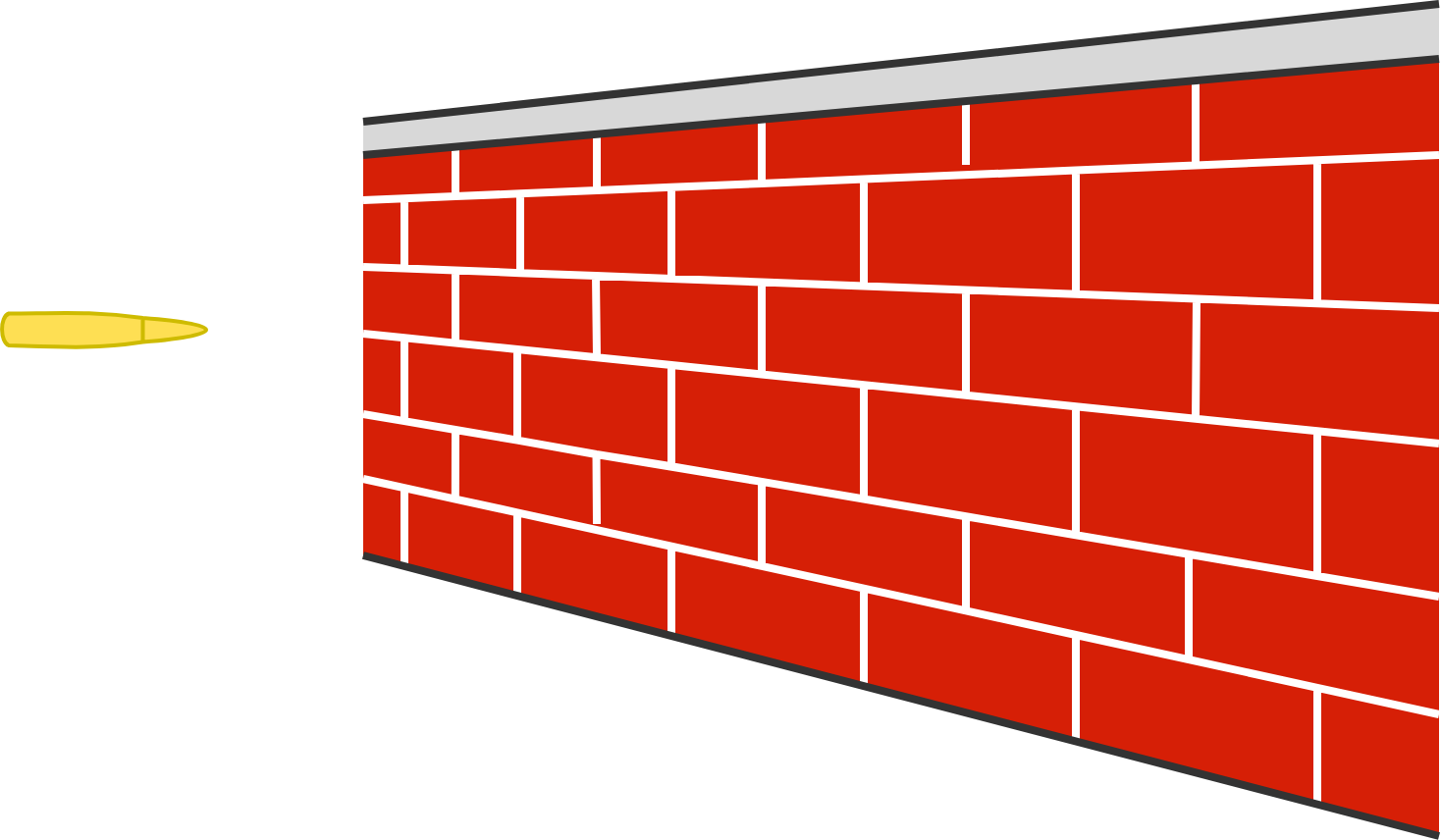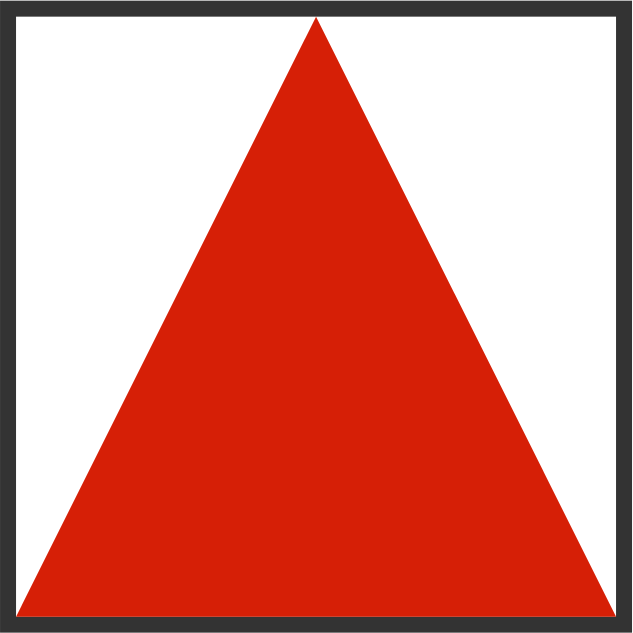# Problems of the Week

Contribute a problem

# 2018-12-17 Basic

A lead bullet is fired and becomes lodged in a brick wall. As the bullet comes to rest, it heats up.

Then, a larger lead bullet is fired at the same wall with the same velocity, and it also becomes lodged in the wall and heats up.

Is the increase in temperature different this time?Assume that all heat from the collision goes into the bullet.

$\large{\begin{array}{ccccc} && & & \square & 3\\ \times & & & & 3 &\square\\ \hline & & & 3 & \square &\square\\ \end{array}}$

Each square in the above multiplication represents a single-digit integer. (Each integer individually may be any value; they do not have to be the same or different.)

What is the final product?

Starting from the number 1, your goal is to get to the number 17 using only these actions:

• multiply by 3.

What is the minimum number of actions it takes to get to 17?

Two soap bubbles of different sizes are joined by a tube.

What will happen next?The triangle in the square below fills half the area of the square. Is it possible to fit a larger triangle entirely within the same square?×